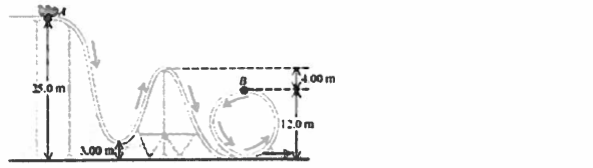Problem: A 240 kg roller coaster car starts from rest at point A and slides down the frictionless loop-the-loop shown in the accompanying figure. Point A is 25.0 m above the ground and point B is 12.0 m above the ground. The height of other points on the track are shown in the diagram.What is the magnitude of the normal force that the track exerts on it at point B?

FREE Expert Solution
96% (138 ratings)
Problem Details

A 240 kg roller coaster car starts from rest at point A and slides down the frictionless loop-the-loop shown in the accompanying figure. Point A is 25.0 m above the ground and point B is 12.0 m above the ground. The height of other points on the track are shown in the diagram.

What is the magnitude of the normal force that the track exerts on it at point B?